## 3D sum or sumif across multiple worksheets in Excel

This tutorial talks about how to sum the same range of cells across multiple consecutive or discontiguous worksheets in Excel.

How to perform a 3D sum across multiple consecutive worksheets?
How to perform a 3D sumif across multiple discontiguous worksheet?

Tip: Before applying the formulas below, make sure that the worksheets that you want to sum should have the same data structure and position as shown in the screenshot below.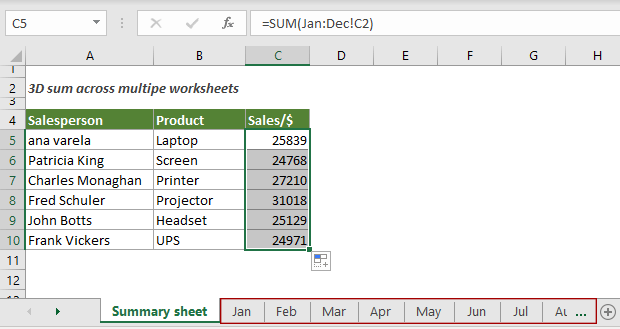#### How to perform a 3D sum across multiple consecutive worksheets?

For the monthly sales workbook shown below, to get a year-end sales summary for each salesperson across all worksheets, you can easily get it done with the SUM function.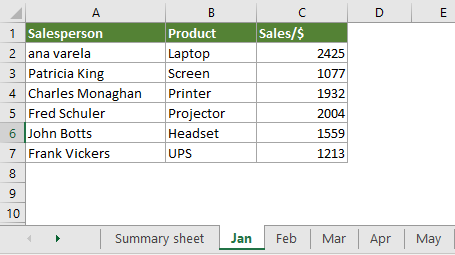Generic Formulas

=SUM(first_sheet:last_sheet!cell)

Arguments

First_sheet: The name of the first worksheet where you want to summarize the data;
Last_sheet: The name of the last worksheet where you want to summarize the data;
Cell: The cell reference which you want to sum the values from.

How to use this formula?

1. Select a cell in the summary worksheet (here I select C5).

2. Copy or enter the below formula into it and press the Enter key. Select the result cell and then drag its AutoFill Handle down to get the sales summary for other salespersons.

=SUM(Jan:Dec!C2)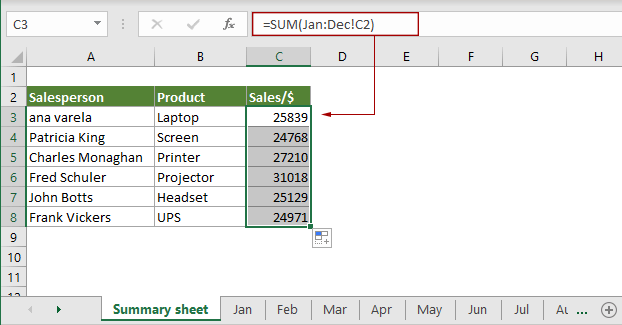Explanation of this formula

=SUM(Jan:Dec!C2): The SUM function sums cell C2 from the worksheet Jan to Dec in the current workbook.

#### How to perform a 3D sumif across multiple discontiguous worksheet?

Take the above workbook as an example, to get a year-end sales summary for each salesperson across discontiguous worksheets such as worksheets Jan, Mar, Apr and Oct, you can combine the SUMPRODUCT, SUMIF and INDIRECT functions to get it down.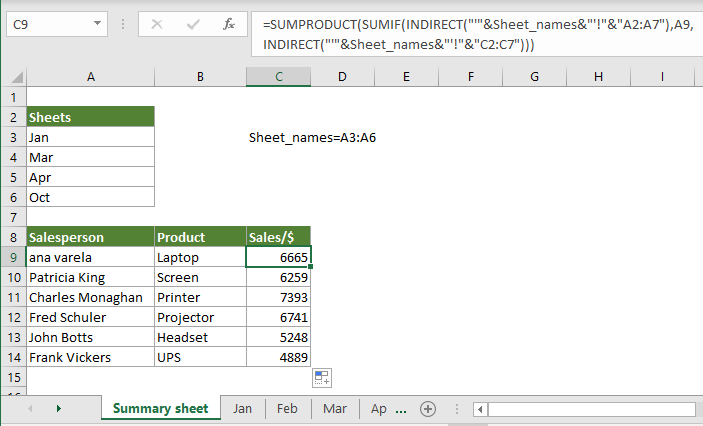Tip: Before applying the formula, you need to list all needed sheet names in the summary worksheet and give a name to the range. In this case, Sheet_names is the name of the range that lists the sheet names.

Generic formula

=SUMPRODUCT(SUMIF(INDIRECT("'"&name_of_sheet_names_range&"'!" & "criteria_range"),criterion,INDIRECT("'"&name_of_sheet_names_range&"'!" &"sum_range")))

Arguments

Name_of_sheet_names_range: It is the range name of the sheet names list;
Criteria_range: The same criteria range in each worksheet that you will sum cells based on;
Criterion: A cell references the criterion in the main worksheet that matches the first value in the criteria_range you will sum cells based on;
Sum_range: The same range of cells in each sheet from which you want to sum the values.

How to use this formula?

1. Select a blank cell in the summary sheet.

2. Copy or enter the below formula into it and press the Enter key. Select the result cell and then drag its AutoFill Handle down to get the sales summary for other salespersons in specified worksheets.

=SUMPRODUCT(SUMIF(INDIRECT("'"&Sheet_names&"'!"&"A2:A7"),A9,INDIRECT("'"&Sheet_names&"'!"&"C2:C7")))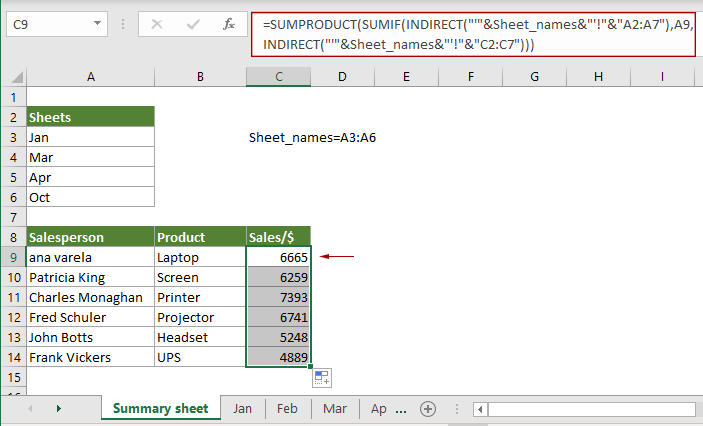#### Related functions

Excel SUM function
The Excel SUM function adds values.

Excel SUMPRODUCT function
The Excel SUMPRODUCT function can be used to multiply two or more columns or arrays together, and then get the sum of products.

Excel SUMIF function
The Excel SUMIF function can help to sum cells based on one criterion

Excel INDIRECT function
The Excel INDIRECT function converts a text string to a valid reference.

#### Related formulas

Calculate running total
This tutorial explains a simple formula to help calculating running total in Excel.

Get subtotal by invoice number
This tutorial explains a formula based on the COUNTIF and SUMIF functions to get subtotal by invoice number in Excel.

Subtotal by colors
This step-by-step guide helps you perform subtotal by color with the SUMIF function.

Two-way summary counting
In this tutorial, we will discuss how to use the COUNTIFS function to create two-way summary count in Excel.

### The Best Office Productivity Tools

#### Kutools for Excel - Helps You To Stand Out From Crowd

Would you like to complete your daily work quickly and perfectly? Kutools for Excel brings 300 powerful advanced features (Combine workbooks, sum by color, split cell contents, convert date, and so on...) and save 80% time for you.

• Designed for 1500 work scenarios, helps you solve 80% Excel problems.
• Reduce thousands of keyboard and mouse clicks every day, relieve your tired eyes and hands.
• Become an Excel expert in 3 minutes. No longer need to remember any painful formulas and VBA codes.
• 30-day unlimited free trial. 60-day money back guarantee. Free upgrade and support for 2 years.#### Office Tab - Enable Tabbed Reading and Editing in Microsoft Office (include Excel)

• One second to switch between dozens of open documents!
• Reduce hundreds of mouse clicks for you every day, say goodbye to mouse hand.
• Increases your productivity by 50% when viewing and editing multiple documents.
• Brings Efficient Tabs to Office (include Excel), Just Like Chrome, Firefox, And New Internet Explorer.